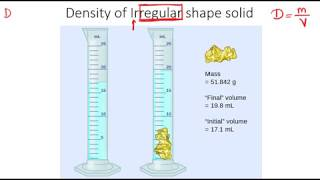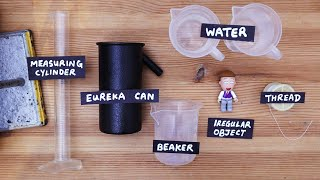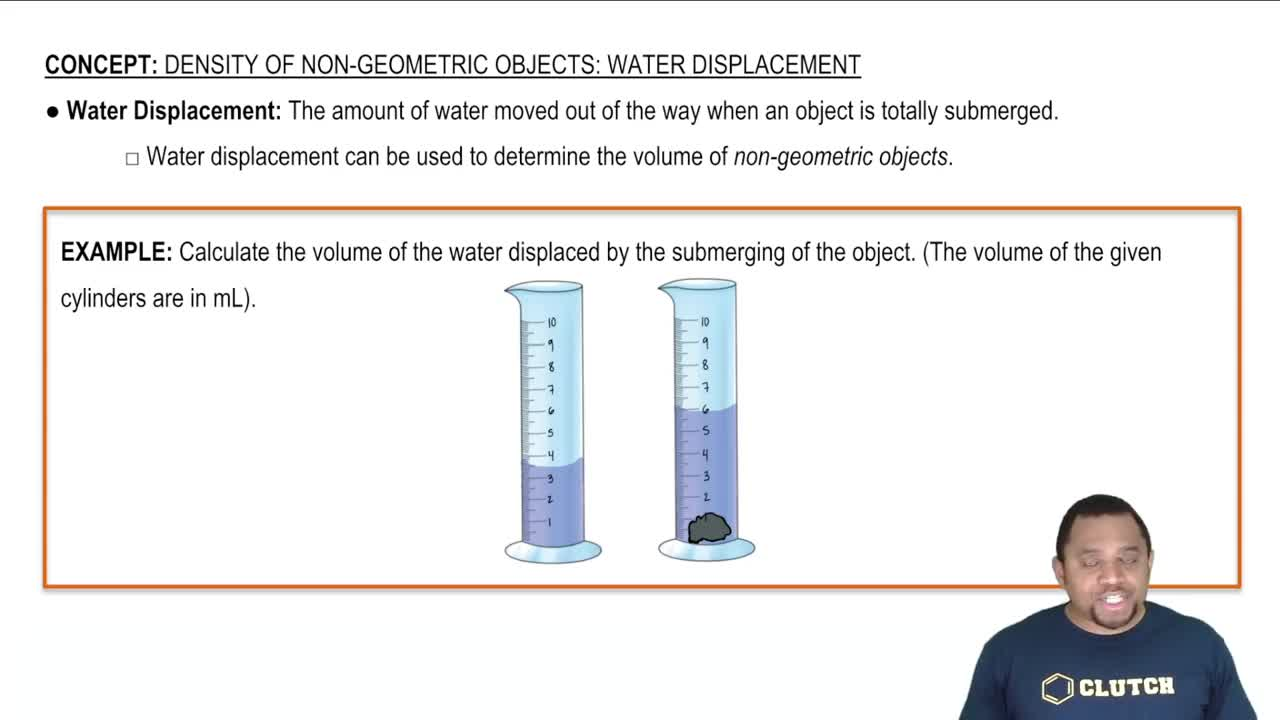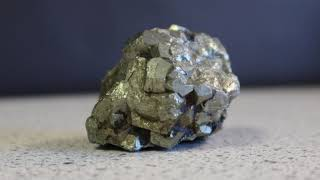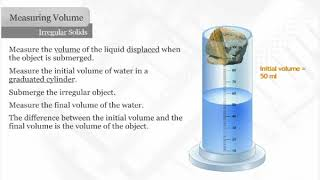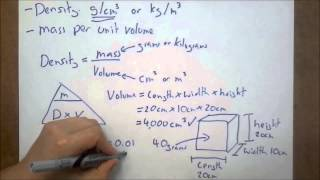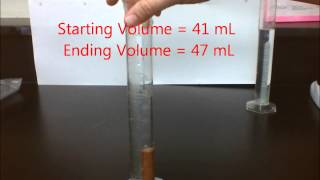Start typing, then use the up and down arrows to select an option from the list.
1. 1. Intro to General Chemistry2. Density of Non-Geometric Objects
Problem

# A supposedly gold nugget displaces 19.3 mL of water and has a mass of 371 g. Could the nugget be made of gold?

Relevant Solution1m
Play a video:
Hey everyone. So here we're told you are given an unknown metal with a weight of 59 g, you drop it in a graduated cylinder with 50 ml of water. They tell us after doing this, the volume of the water became 57.50 mm determine the identity of the metal here were given medals, each with their own density. Remember density equals mass over volume. We know the unknown metal weighs 59 g. Here this is a water displacement question. All we have to do to figure out the volume of the unknown is take the volume after adding it to water minus the volume of the water before we even place the unknown object into it. So this comes out to 7.50 ml difference, that difference in water volume is because of that unknown metal. So that's its volume. So when we do this, we get an answer off 7.87 grams per milliliter, which is the same thing as grams per centimeters cubed. When we look the metal with the density closest to this answer is option C. Iron Iron also has a density of 7.87. So C would be our final answer